# Tits building

A building is a pair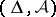consisting of a simplicial complexand a familyof subcomplexes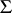(appartments) satisfying the following conditions: i) each simplex ofor of any appartmentis contained in a maximal simplex (a chamber), and each chamber oforhas the same finite dimension(the rank of the building); ii) each appartmentis connected, in the sense that for any two chambers,inthere is a sequence of chambers ofstarting withand ending with, the intersection of any two successive members of which is an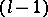-simplex; iii) any-simplex of(respectively, of any appartment) is contained in more thanchambers of(respectively, in exactlychambers of); iv) any two chambers,ofare contained in some appartment; and v) if two simplices,ofare contained in two appartments,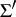, then there is an isomorphism fromontofixing bothandpointwise.

### Example 1.

Letbe a vector space, letconsist of all chains of non-zero subspaces of(ordered by inclusion), and let each appartment consist of all those chains consisting of subspaces spanned by the non-empty subsets of some basis of. Then, together with these subcomplexes, is a building.

### Example 2.

More generally, letbe a group with a Tits system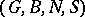, letbe the disjoint union of all the cosets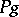,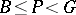,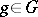, ordered by reverse inclusion, and let appartments be the sets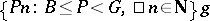,. Then, equipped with these appartments, is a building whose rank is that of the Tits system. Moreover, in this situationis transitive on the pairs consisting of an appartment and a chamber of that appartment.

Ifis a building, then all appartments are isomorphic to the simplicial complex determined by a Coxeter group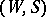— the Weyl group of the building, and unique up to isomorphism — as follows: simplices are the right cosets of the subgroups generated by non-empty subsets of, where cosets are again ordered by reverse inclusion. In Example 2 this Weyl group is the same as that of the Tits system. A building is called spherical or affine if its Weyl group is. Affine buildings of rankare just trees in which each vertex is adjacent to at leastothers.

Buildings are geometric or combinatorial versions of Tits systems. The most important buildings arise from Tits systems associated with algebraic groups (cf. Algebraic group), although not all buildings do. The two main theorems concerning buildings are classification theorems due to J. Tits [a4], [a5]: 1) any spherical building of rank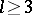having an indecomposable Weyl group is isomorphic to the building determined by the Tits system of a simple algebraic group; and 2) ifis an affine building of rank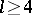having an indecomposable Weyl group, and if each-simplex is contained in a finite number of chambers, thenis isomorphic to the complex determined by the affine Tits system of a simple algebraic group over a complete local field. These results stem from the fact that a great deal of geometric information is encoded in the building axioms. In fact, 1) can be viewed as a vast generalization of the classical result that a suitable axiomatization of the notion of projective space leads to a classification in terms of the standard vector space model (cf. Example 1). By contrast, spherical buildings of rank, and affine buildings of rank, are too wild to be classifiable: there are many constructions, including free ones. Moreover, spherical buildings of rankwith as Weyl group the symmetric group onletters correspond naturally to projective planes (incident point-line pairs of a plane corresponding to chambers, cf. also Projective plane).

Buildings are important for the study of the internal structure, representation theory and geometry of simple algebraic groups. They play important roles in the study of finite simple groups and finite geometries as well as in various cohomological questions.